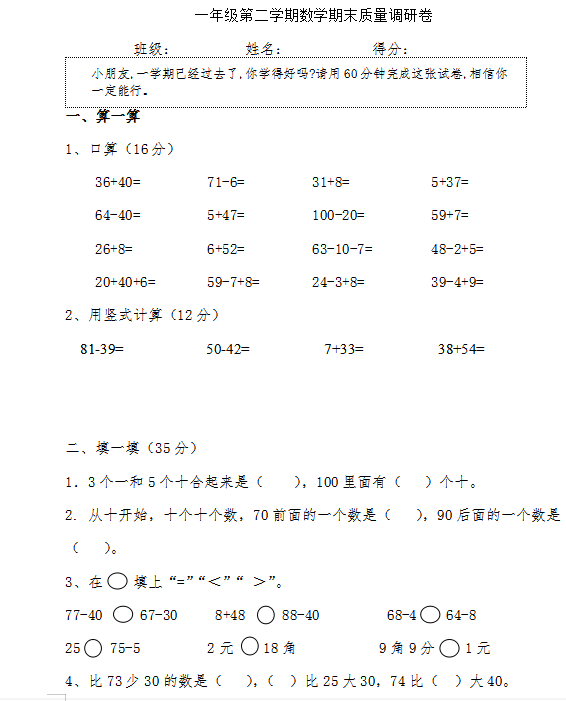1、口算（16分）

36+40=         71-6=         31+8=           5+37=

64-40=         5+47=         100-20=         59+7=

26+8=          6+52=         63-10-7=        48-2+5=

20+40+6=       59-7+8=       24-3+8=         39-4+9=• 终生VIP优惠券
50

满100元可用

仅限购买永久VIP使用

有效期至2023-10-15 10:37

• 20元通用优惠券
20

满50元可用

仅限购买VIP使用

有效期至2023-10-06 11:01